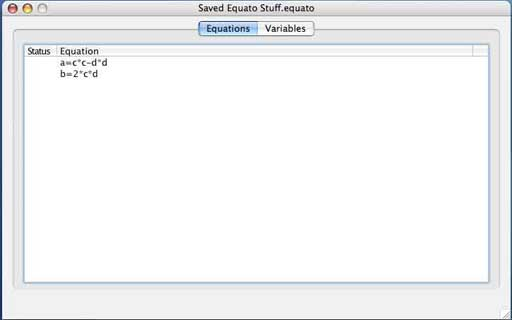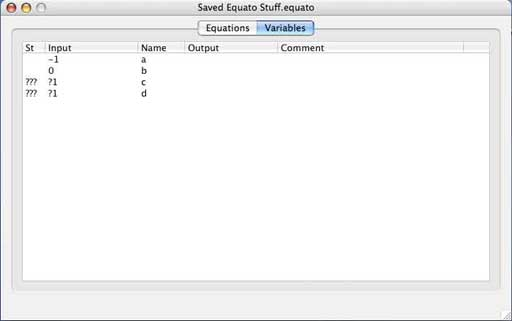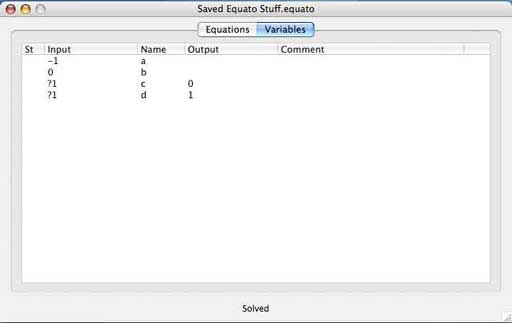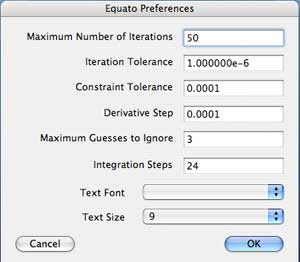## Equato - An Equation Solver

Equato is a relatively simple equation solver that works using constraint propagation and Newton Raphson iteration. It takes a set of simple algebraic equations and a list of input variables and produces a list of output variables. You can invoke an iterative solution by taking a guess for an unknown value. The program is flakey and incomplete, and relies on a solution technique of limited reliability. On the other hand, it is free, it runs on the Macintosh and possible on Windows, and it sometimes produces useful answers.The Equation Pane There are two panes in Equato, one for equations and one for variables. You click and edit an equation as you might enter values in a single column spreadsheet. The equations may contain variables (with any reasonable number of letters or numbers in their name) and may use the operators +, -, *, /, ^ for exponentiation, sin, cos, atan of two arguments, exp and ln. You can enter comments alone or after an equation line by preceding them with an apostrophe.The Variable Pane The variable pane shows the variables, their status, their input value or guess value, their computed output value and, if you care to enter one, a descriptive comment. The ??? in the status column means that the values have not been solved for. The ?1 specifies a guess value of 1 for the variable and indicates that an iterative solution should be used if necessary. The solver first tries simple constraint relaxation. This works surprisingly often. If it fails, it shows a lot of question marks, unless the problem is overdetermined in which case see above. If there are any guess values it formulates the problem as having N equations and N unknowns, or at least tries to, and then uses a gradient following algorithm, Newton Raphson, to iterate towards an answer. If this doesn't work, it tries working with some subset of the guesses specified in hopes of finding a stable approach to an answer. As you can see in this example, it has computed the square root of minus one to be i.Preferences If Equato is still causing trouble, or you'd like to try your luck at getting some more accuracy out of it, try tweaking the preferences. You can try more iterations, a tighter iteration tolerance, or you can try relaxing the constraint tolerance and adding some more equations, or even dink around with the step size used for approximating the derivatives. Newton-Raphson is actually a pretty stable algorithm if you restrict the functions you use. The maximum guesses to ignore is useful for cases which are sometimes unstable, but could be stable if you weren't using so many iteration variables. For simple problems, Equato can be pretty fast, but if you are clever with the preferences you can probably get it to explode combinatorically. The integration steps are the number of steps to use for Simpson's rule when evaluating expressions containing integral(f(x), x, a, b). This should be even, but Equato will add one if it is odd. The text font and size stuff might be useful, but it is poorly implemented. The default font is the system font, and the default size should work with the tables. Changing either of these might make things look "pretty".